xTurn (geometry)Encyclopedia
A turn is an angle
Angle
In geometry, an angle is the figure formed by two rays sharing a common endpoint, called the vertex of the angle.Angles are usually presumed to be in a Euclidean plane with the circle taken for standard with regard to direction. In fact, an angle is frequently viewed as a measure of an circular arc...

equal to a 360°
Degree (angle)
A degree , usually denoted by ° , is a measurement of plane angle, representing 1⁄360 of a full rotation; one degree is equivalent to π/180 radians...

or 2
Pi
' is a mathematical constant that is the ratio of any circle's circumference to its diameter. is approximately equal to 3.14. Many formulae in mathematics, science, and engineering involve , which makes it one of the most important mathematical constants...

radian
Radian
Radian is the ratio between the length of an arc and its radius. The radian is the standard unit of angular measure, used in many areas of mathematics. The unit was formerly a SI supplementary unit, but this category was abolished in 1995 and the radian is now considered a SI derived unit...

s orTau (2π)
Tau is a mathematical constant equal to the ratio of any circle's circumference to its radius, and has a value of approximately 6.28318531. This number also appears in many common formulas, often because it is the period of some very common functions — sine, cosine, , and others that involve...

(tau) radians. A turn is also referred to as a revolution or complete rotation or full circle or cycle or rev or rot.

A turn can be subdivided in many different ways, into half turns, quarter turns, centiturns, milliturns, binary angles, points etc.

## Subdivision of turns

A turn can be divided in 100 centiturns or 1000 milliturns with each milliturn corresponding to an angle
Angle
In geometry, an angle is the figure formed by two rays sharing a common endpoint, called the vertex of the angle.Angles are usually presumed to be in a Euclidean plane with the circle taken for standard with regard to direction. In fact, an angle is frequently viewed as a measure of an circular arc...

of 0.36°, which can also be written as 21'36".

Binary fractions of a turn are also used. Sailors have traditionally divided a turn into 32 points
Compass Point
Compass Point may refer to:* Compass point, a direction on a traditional compass* Compass Point * Compass Point Shopping Centre, a shopping mall in Singapore* Compass Point Studios, a studio in Nassau, Bahamas...

. The binary degree, also known as the binary radian (or brad), is 1/256 turn. The binary degree is used in computing so that an angle can be efficiently represented in a single byte
Byte
The byte is a unit of digital information in computing and telecommunications that most commonly consists of eight bits. Historically, a byte was the number of bits used to encode a single character of text in a computer and for this reason it is the basic addressable element in many computer...

(albeit to limited precision). Other measures of angle used in computing may be based on dividing one whole turn into 2n equal parts for other values of n.

The notion of turn is commonly used for planar rotations. Two special rotations have acquired appellations of their own: a rotation through 180° is commonly referred to as a half-turn (radians), a rotation through 90° is referred to as a quarter-turn. A half-turn is often referred to as a reflection in a point since these are identical for transformations in two-dimensions.

## History

The word turn originates via Latin and French from the Greek word τόρνος (tornos – a lathe
Lathe
A lathe is a machine tool which rotates the workpiece on its axis to perform various operations such as cutting, sanding, knurling, drilling, or deformation with tools that are applied to the workpiece to create an object which has symmetry about an axis of rotation.Lathes are used in woodturning,...

).

The geometric notion of a turn has its origin in the sailors terminology of knots where a turn
Turn (knot)
A turn is one round of rope on a pin or cleat, or one round of a coil. Turns can be made around various objects, through rings, or around the standing part of the rope itself or another rope. A turn also denotes a component of a knot....

means one round of rope on a pin or cleat
Cleat
Cleat may refer to:* Cleat , a type or part of a shoe* Cleat , a fitting on ships, boats, and docks to which ropes are tied* Cleats , a comic strip by Bill Hinds...

, or one round of a coil
Coil
A coil is a series of loops. A coiled coil is a structure in which the coil itself is in turn also looping.-Electromagnetic coils:An electromagnetic coil is formed when a conductor is wound around a core or form to create an inductor or electromagnet...

. For knots the English terms of single turn, round turn and double round turn do not translate directly into the geometric notion of turn, but in German the correspondence is exact.

In 1697 David Gregory used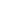(pi/rho) to denote the perimeter of a circle (i.e. the circumference) divided by its radius, though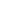(delta/pi) had been used by William Oughtred
William Oughtred
William Oughtred was an English mathematician.After John Napier invented logarithms, and Edmund Gunter created the logarithmic scales upon which slide rules are based, it was Oughtred who first used two such scales sliding by one another to perform direct multiplication and division; and he is...

in 1647 for the ratio of diameter to perimeter. The first use ofon its own with its present meaning of perimeter/diameter was by William Jones in 1706. Euler adopted the symbol with that meaning in 1737, leading to its widespread use.

The idea of using centiturns and milliturns as units was introduced by Sir Fred Hoyle.

Robert Palais proposed in 2001 to use the number of radians in a turn as the fundamental circle constant instead of, in order to make mathematics simpler and more intuitive, using a "pi with three legs" symbol to denote 1 turn (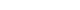). In 2010, Michael Hartl proposed to use the Greek letterTau (2π)
Tau is a mathematical constant equal to the ratio of any circle's circumference to its radius, and has a value of approximately 6.28318531. This number also appears in many common formulas, often because it is the period of some very common functions — sine, cosine, , and others that involve...

(tau) to represent the number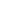Tau (2π)
Tau is a mathematical constant equal to the ratio of any circle's circumference to its radius, and has a value of approximately 6.28318531. This number also appears in many common formulas, often because it is the period of some very common functions — sine, cosine, , and others that involve...

instead.

## Mathematical constants

One turn can be identified with (≈6.28) radians.

Similarly, half a turn is often identified with the mathematical constantbecause half a turn is(≈3.14) radians.

## Conversion of some common angles

Units | Values
Turns   0 1/12 1/10 1/8 1/6 1/5 1/4 1/2 3/4 1
Radian
Radian
Radian is the ratio between the length of an arc and its radius. The radian is the standard unit of angular measure, used in many areas of mathematics. The unit was formerly a SI supplementary unit, but this category was abolished in 1995 and the radian is now considered a SI derived unit...

s
0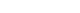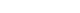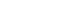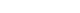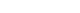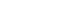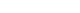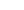Degrees
Degree (angle)
A degree , usually denoted by ° , is a measurement of plane angle, representing 1⁄360 of a full rotation; one degree is equivalent to π/180 radians...

30° 36° 45° 60° 72° 90° 180° 270° 360°
Grads
Grad (angle)
The gradian is a unit of plane angle, equivalent to of a turn. It is also known as gon, grad, or grade . One grad equals of a degree or of a radian...

0g 33⅓g 40g 50g 66⅔g 80g 100g 200g 300g 400g

## Examples of use

• As an angular unit it is particularly useful for large angles, such as in connection with coil
Coil
A coil is a series of loops. A coiled coil is a structure in which the coil itself is in turn also looping.-Electromagnetic coils:An electromagnetic coil is formed when a conductor is wound around a core or form to create an inductor or electromagnet...

s and rotating
Rotation
A rotation is a circular movement of an object around a center of rotation. A three-dimensional object rotates always around an imaginary line called a rotation axis. If the axis is within the body, and passes through its center of mass the body is said to rotate upon itself, or spin. A rotation...

objects. See also winding number
Winding number
In mathematics, the winding number of a closed curve in the plane around a given point is an integer representing the total number of times that curve travels counterclockwise around the point...

.
• Turn is used in complex dynamics
Complex dynamics
Complex dynamics is the study of dynamical systems defined by iteration of functions on complex number spaces. Complex analytic dynamics is the study of the dynamics of specifically analytic functions.-Techniques:*General** Montel's theorem...

for measure of external
External ray
An external ray is a curve that runs from infinity toward a Julia or Mandelbrot set.This curve is only sometimes a half-line but is called ray because it is image of ray....

and internal angles. The sum of external angles of a polygon equals one turn.
• Pie chart
Pie chart
A pie chart is a circular chart divided into sectors, illustrating proportion. In a pie chart, the arc length of each sector , is proportional to the quantity it represents. When angles are measured with 1 turn as unit then a number of percent is identified with the same number of centiturns...

s illustrate proportions of a whole as fractions of a turn. Each one percent is shown as an angle of one centiturn.

## Kinematics of turns

In kinematics
Kinematics
Kinematics is the branch of classical mechanics that describes the motion of bodies and systems without consideration of the forces that cause the motion....

a turn is a rotation less than a full revolution.
A turn may be represented in a mathematical model
Mathematical model
A mathematical model is a description of a system using mathematical concepts and language. The process of developing a mathematical model is termed mathematical modeling. Mathematical models are used not only in the natural sciences and engineering disciplines A mathematical model is a...

that uses expressions of complex number
Complex number
A complex number is a number consisting of a real part and an imaginary part. Complex numbers extend the idea of the one-dimensional number line to the two-dimensional complex plane by using the number line for the real part and adding a vertical axis to plot the imaginary part...

s or quaternion
Quaternion
In mathematics, the quaternions are a number system that extends the complex numbers. They were first described by Irish mathematician Sir William Rowan Hamilton in 1843 and applied to mechanics in three-dimensional space...

s. In the complex plane
Complex plane
In mathematics, the complex plane or z-plane is a geometric representation of the complex numbers established by the real axis and the orthogonal imaginary axis...

every non-zero number has a polar coordinate expression z = r cos a + r i sin a where r > 0 and a is in [0, 2π).
A turn of the complex plane arises from multiplying z = x + i y by an element u = eb i that lies on the unit circle
Unit circle
In mathematics, a unit circle is a circle with a radius of one. Frequently, especially in trigonometry, "the" unit circle is the circle of radius one centered at the origin in the Cartesian coordinate system in the Euclidean plane...

:Frank Morley
Frank Morley
Frank Morley was a leading mathematician, known mostly for his teaching and research in the fields of algebra and geometry...

consistently referred to elements of the unit circle as turns in the book Inversive Geomety (1933) that he coauthored with his son Frank Vigor Morley.

The Latin term for turn is versor, which is a quaternion that can be visualized as an arc
Arc (geometry)
In geometry, an arc is a closed segment of a differentiable curve in the two-dimensional plane; for example, a circular arc is a segment of the circumference of a circle...

of a great circle
Great circle
A great circle, also known as a Riemannian circle, of a sphere is the intersection of the sphere and a plane which passes through the center point of the sphere, as opposed to a general circle of a sphere where the plane is not required to pass through the center...

. The product of two versors can be compared to a spherical triangle where two sides add to the third. For the kinematics of rotation in three dimensions, see quaternions and spatial rotation
Quaternions and spatial rotation
Unit quaternions provide a convenient mathematical notation for representing orientations and rotations of objects in three dimensions. Compared to Euler angles they are simpler to compose and avoid the problem of gimbal lock. Compared to rotation matrices they are more numerically stable and may...

.

## External links

The source of this article is wikipedia, the free encyclopedia.  The text of this article is licensed under the GFDL.